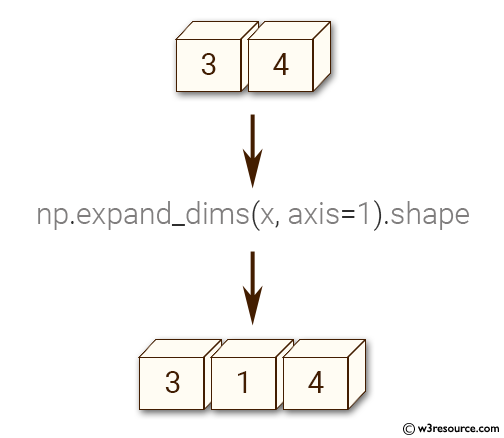﻿ NumPy: Insert a new axis within a 2-D array - w3resource# NumPy: Insert a new axis within a 2-D array

## NumPy: Array Object Exercise-56 with Solution

Write a NumPy program to insert a new axis within a 2-D array.
2-D array of shape (3, 4).
New shape will be will be (3, 1, 4).

Pictorial Presentation:Sample Solution:-

Python Code:

``````import numpy as np
x = np.zeros((3, 4))
y = np.expand_dims(x, axis=1).shape
print(y)
```
```

Sample Output:

```(3, 1, 4)
```

Python Code Editor:

Have another way to solve this solution? Contribute your code (and comments) through Disqus.

What is the difficulty level of this exercise?

Test your Python skills with w3resource's quiz

﻿

## Python: Tips of the Day

List comprehension:

```>>> m = [x ** 2 for x in range(5)]
>>> m
[0, 1, 4, 9, 16]
```